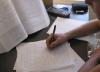# 2.2.5 - Measures of Spread

The standard deviation is the most commonly used measure of variability when working with interval- or ratio-level variables. In a sample, this is denoted as $$s$$. In a population, we use the Greek letter $$\sigma$$ ("sigma").

When computing the standard deviation by hand, it is necessary to first compute the variance. The variance is equal to the standard deviation squared. In a sample, this is denoted as $$s^2$$. In a population, it is $$\sigma^2$$.

On this page we will provide some examples of computing standard deviation and variance by hand, but after this lesson you will always compute the standard deviation and variance using software such as Minitab.

Sample Standard Deviation
$$s=\sqrt{\dfrac{\sum (x-\overline{x})^{2}}{n-1}}$$

There are a number of methods for calculating the standard deviation. If you look through different textbooks or search online, you may find different formulas and procedures. To compute the standard deviation for a sample, we will use the formulas above and the following steps:

Step 1: Compute the sample mean: $$\overline{x} = \dfrac{\sum x}{n}$$.

Step 2: Subtract the sample mean from each individual value: $$x-\overline{x}$$, these are the deviations.

Step 3: Square each deviation: $$(x-\overline{x})^{2}$$, these are the squared deviations.

Step 4: Add the squared deviations: $$\sum (x-\overline{x})^{2}$$, this is the sum of squares.

Step 5: Divide the sum of squares by $$n-1$$: $$\frac{\sum (x-\overline{x})^{2}}{n-1}$$, this is the sample variance $$(s^{2})$$.

Step 6: Take the square root of the sample variance: $$\sqrt{\frac{\sum (x-\overline{x})^{2}}{n-1}}$$, this is the sample standard deviation ($$s$$).

The formula for computing the standard deviation in a population is slightly different. Note that the denominator chances from $$n-1$$ to $$N$$. In this course, we will primarily be using the sample standard deviation, but you can review the following formulas to see the similarities.

Population Standard Deviation
$$\sigma=\sqrt{\dfrac{\sum (x-\mu)^{2}}{N}}$$
Sample Variance
$$s^2=\dfrac{\sum (x-\overline{x})^{2}}{n-1}$$
Population Variance
$$\sigma^2=\dfrac{\sum (x-\mu)^{2}}{N}$$

## Video Example

The video below walks through an example of computing a sample standard deviation by hand.

## Example: Hours Spent StudyingA professor asks a sample of 7 students how many hours they spent studying for the final. Their responses are: 5, 7, 8, 9, 9, 11, and 13.

Step 1: Compute the mean

$$\overline{x} = \dfrac{\sum x}{n}=\dfrac{5+7+8+9+9+11+13}{7}=8.857$$

Step 2: Compute the deviations

$$x$$ $$x - \overline{x}$$
5 $$5 - 8.857 = -3.857$$
7 $$7 - 8.857 = -1.857$$
8 $$8 - 8.857 = -0.857$$
9 $$9 - 8.857 = 0.143$$
9 $$9 - 8.857 = 0.143$$
11 $$11 - 8.857 = 2.143$$
13 $$13 - 8.857 = 4.143$$

Step 3: Square the deviations

$$x$$ $$x - \overline{x}$$ $$(x-\overline{x})^{2}$$
5 $$5 - 8.857 = -3.857$$ $$-3.857^{2} = 14.876$$
7 $$7 - 8.857 = -1.857$$ $$-1.857^{2} = 3.448$$
8 $$8 - 8.857 = -0.857$$ $$-0.857^{2} = 0.734$$
9 $$9 - 8.857 = 0.143$$ $$0.143^{2} = 0.0020$$
9 $$9 - 8.857 = 0.143$$ $$0.143^{2} = 0.0020$$
11 $$11 - 8.857 = 2.143$$ $$2.143^{2} = 4.592$$
13 $$13 - 8.857 = 4.143$$ $$4.143^{2} = 17.164$$

Step 4: Sum the squared deviations

$$SS=\sum (x-\overline{x})^{2}=14.876+3.448+0.734+.020+.020+4.592+17.164=40.854$$

The sum of squares is 40.854

Step 5: Divide by n - 1 to compute the variance

$$s^{2}=\dfrac{\sum (x-\overline{x})^{2}}{n-1}=\dfrac{40.854}{7-1}=6.809$$

The variance is 6.809

Step 6: Take the square root of the variance

$$s=\sqrt{s^{2}}=\sqrt{6.809}=2.609$$

The standard deviation is 2.609

  Link ↥ Has Tooltip/Popover Toggleable Visibility